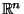## Bolzano-Weierstraß Theorem

Every Bounded infinite set inhas an Accumulation Point. For, the theorem can be stated as follows: If a Set in a Metric Space, finite-dimensional Euclidean Space, or First-Countable Space has infinitely many members within a finite interval, then it has at least one Limit Pointsuch that. The theorem can be used to prove the Intermediate Value Theorem.